# Availability & Irreversibility Notes | Study Thermodynamics - Mechanical Engineering

## Mechanical Engineering: Availability & Irreversibility Notes | Study Thermodynamics - Mechanical Engineering

The document Availability & Irreversibility Notes | Study Thermodynamics - Mechanical Engineering is a part of the Mechanical Engineering Course Thermodynamics.
All you need of Mechanical Engineering at this link: Mechanical Engineering

Introduction

Availability: When a system is subjected to a process from its original state to dead state the maximum amount of useful work that can be achieved under ideal conditions is known as available energy or availability of the system.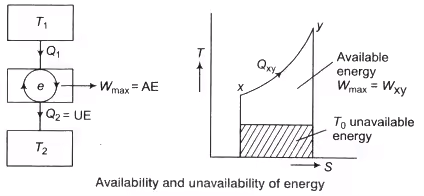Wmax = AE = Qxy – T0(Sy-Sx)
Unavailable Energy:  UAE = T0(Sy-Sx)
where, Sx and Sy are the entropy at x and y, respectively.
The Available Energy (AE) is also known as exergy and the Unavailable Energy (UAE) as anergy. The energy which cannot be utilised for doing useful work is called unavailable energy. Irreversibility is equivalent to energy destroyed, hence also known as energy destruction consider the example given below.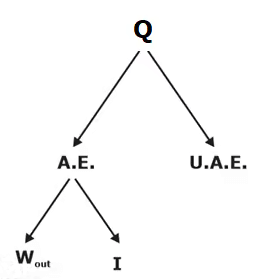Decrease in Availability When Heat Transfer Through Finite Temperature Difference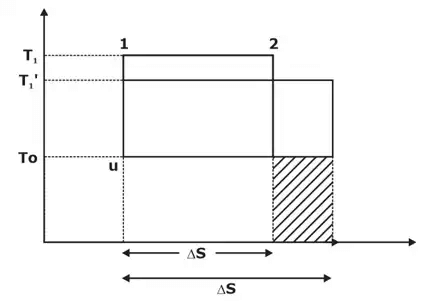Consider a reversible heat engine operating between T1 and T0.
Q1 = T1. Δs
W = AE = (T1 – T0)Δs
Let us now consider heat Q1 is transferred through a finite temperature difference.
Q1 = T1 Δs = T’1 . Δs’
Δs’ > Δs
Q2 = T0 Δs → Initial UAE
Q’2 = T0 Δs’ ⇒ Afterward UAE
Q’2 > Q2
W’ =Q’1 – Q’2
W’ = T’1 Δs’ – T0Δs’
W’ = (T’1 – T0) Δs’
W = (T1 – T0) Δs
Hence increase in UAE and the shaded portion represent increase in UAE.

Second Law Efficiency

Second law efficiency is the ratio of the exergy recovered to the exergy spent. OR It is the ratio of actual work produced to the max work produced under reversible condition. Consider the case of heat engine.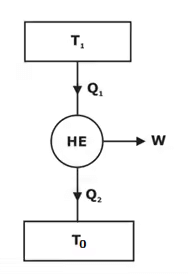Energy recovered = Q1 - Q2 = W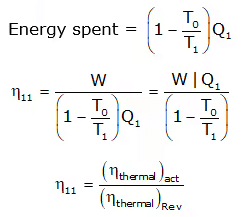The second law efficiency is measure of the performance of a device relative to its performance under reversible conditions.

Exergy of a Closed System

Consider a piston cylinder device that contains a fluid of mass m at temperature T and pressure P. The system is then allowed to undergo a differential change of state during which volume changes by dV and heat  is transferred from the system to surroundings.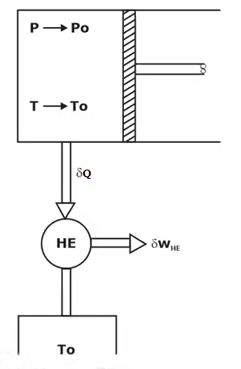For change of exergy from state 1 to state 2.
Φ1 = (E1 – E0) + P0(V1 – V0) – T0(S1 – S0)
Φ2 = (E2 – E0) + P0(V2 – V0) – T0(S2 – S0)
Φ1 – ϕ2 = Energy at state 1 – Energy at state 2
Φ1 – ϕ2 = (E1 – E2) + P0 (V1 – V2) – T0(S1 – S2)

Exergy of Open System
A flowing fluid has an additional form of energy, called flow energy, which is the energy needed to maintain flow in Pipe.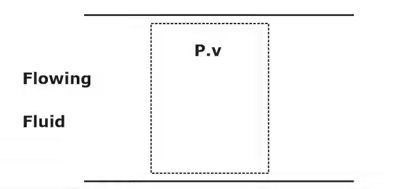Exergy associated with system is
Φflowing fluid = ϕnon flow fluid + ϕflow
Φflowing fluid = (E1 – E0) + P0(V1 – V0) – T0(S1 – S0) + P1V1 – P0V0
Φflowing = U- U0 + (KE)1 - (KE)0 + (PE)1 – (PE)0 + P1V1 – P0V0 – T0 (S1 – S0)
Φflowing fluid = (U1 + P1V1) – (U0 + P0V0) – T0(S1 – S0) + (KE)1 – (KE)0 + (PE)1 – (PE)0
Φflowing = (H1 – H0) – T0 (S1 – S0) + (KE)1 – (KE)0 + (PE)1 – (PE)2

The document Availability & Irreversibility Notes | Study Thermodynamics - Mechanical Engineering is a part of the Mechanical Engineering Course Thermodynamics.
All you need of Mechanical Engineering at this link: Mechanical EngineeringUse Code STAYHOME200 and get INR 200 additional OFF

## Thermodynamics

33 videos|54 docs|36 tests

Track your progress, build streaks, highlight & save important lessons and more!

,

,

,

,

,

,

,

,

,

,

,

,

,

,

,

,

,

,

,

,

,

;